# SQL MAX() function

## MAX() function

The aggregate function SQL MAX() is used to find the maximum value or highest value of a certain column or expression. This function is useful to determine the largest of all selected values of a column.

Syntax:

```MAX ([ALL | DISTINCT] expression )
```

DB2 and Oracle Syntax:

```MAX ([ALL | DISTINCT] expression ) OVER (window_clause)
```

Parameters:

Name Description
ALL Applies to all values.
DISTINCT Consider each unique value. DISTINCT is not meaningful with MAX function.
expression Expression made up of a single constant, variable, scalar function, or column name or any combination of arithmetic, bitwise, and string operators. MAX can be used with numeric, character, and datetime columns, but not with bit columns. Aggregate functions and subqueries are not permitted.

DBMS Support: COUNT() function

 DBMS Command MySQL Supported PostgreSQL Supported SQL Server Supported Oracle Supported

Syntax diagram - MAX() function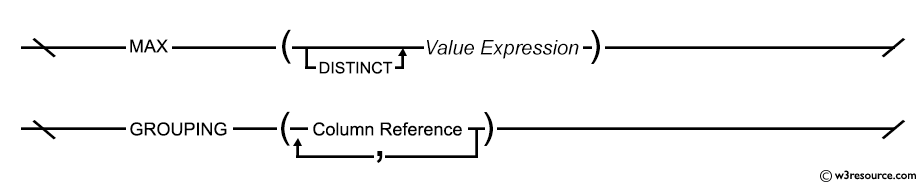Example:

To get maximum 'ord_amout' from the 'orders' table, the following SQL statement can be used :

Sample table: orders

``````SELECT MAX (ord_amount)
FROM orders;
``````

Relational Algebra Expression: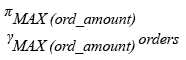Relational Algebra Tree: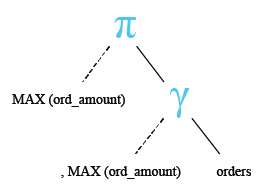Output:

```MAX(ORD_AMOUNT)
---------------
4500
```

Pictorial Presentation: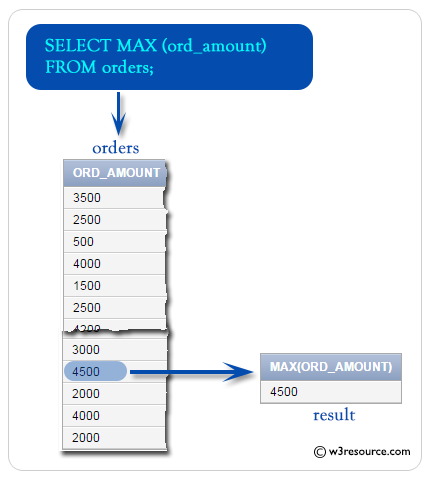## SQL MAX() with addition of two columns

To get the maximum value of (opening_amt+receive_amt) from the customer table, the following SQL statement can be used :

Sample table: customer

``````SELECT MAX (opening_amt+receive_amt)
FROM customer;
``````

Relational Algebra Expression: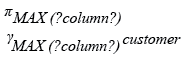Relational Algebra Tree: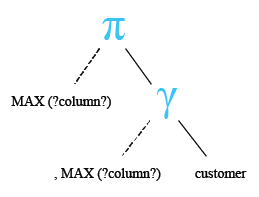Output:

```MAX(OPENING_AMT+RECEIVE_AMT)
----------------------------
19000
```

Note: Outputs of the said SQL statement shown here is taken by using Oracle Database 10g Express Edition

Here is a slide presentation of all aggregate functions.

Practice SQL Exercises

Previous: Avg with round, group by
Next: Max with Group by, Order by

﻿

`select convert(varchar(10), '2011-02-25 21:17:33.933', 120)`